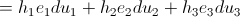## Pages

### 8. Metric Tensor

Measurement in spacetime begins with distance. We have to declare some rules of distances, so called axioms. Let us call $d()$ distance function, that accepts two points that accepts two points as parameters, and the rules are:
Distance of a point $a$ from itself equals zero:
$d(a,a)=0$

Distance of a point from $a$ to $b$ is equal to the distance of point $b$ to $a$:
$d(a,b)$= $d(b,a)$

Let us now consider a curvilinear co-ordinate system $(u_{1},u_{2},...u_{n})$. Let $\vec{r}$ be any position vector of the curvilinear system. We then have that $\vec{r}=\vec{r}(u_{1},u_{2},...u_{n})$. Now, from the vector differential calculus we have the differential element $d(\vec{r})$ as equal to the following;
(Nb: we are considering for the case of $n=3$);Here, $e_{1},e_{2}, e_{3}...$ are the direction vector of the unit tangent vectors of the respective coordinate, "$h_{n}$" is simply the scale factor(magnitude) of the tangent vector.
Now, the differential elements of the arc length in general curvilinear system is defined to be,
More elegantly,
Here, we have made use of the Einstein's summation convention. Einstein summation convention is just a notational convention that implies summation over a set of indexed terms in a formula, thus achieving notational brevity . This then, is the equation of the arc length of the curvilinear coordinate system. Here,$g_{\alpha }=\alpha_{p} \cdot \alpha_{q}$ is called the metric coefficient and $\alpha_{n}$ the tangent vector (not necessarily the unit vector) of the concerned axes in a curvilinear coordinates system. The value of $g_{pq}$ are different in different curvilinear coordinate system. In fact, we can say that the metric tensor of any particular coordinate system is the signature of the system concerned.

The tensor $g_{pq}$ satisfies the following property:
(that is, it is symmetric) because the multiplication in the Einstein summation is ordinary multiplication and hence commutative.